Question

# Three resistors with values of 7.0 Ω , 14 Ω , and 20 Ω are connected...

Three resistors with values of 7.0 Ω , 14 Ω , and 20 Ω are connected in series in a circuit with a 9.0 V battery.

A) What is the total equivalent resistance?

B) What is the current in each resistor?

C) At what rate is energy delivered to the 20 Ω resistor?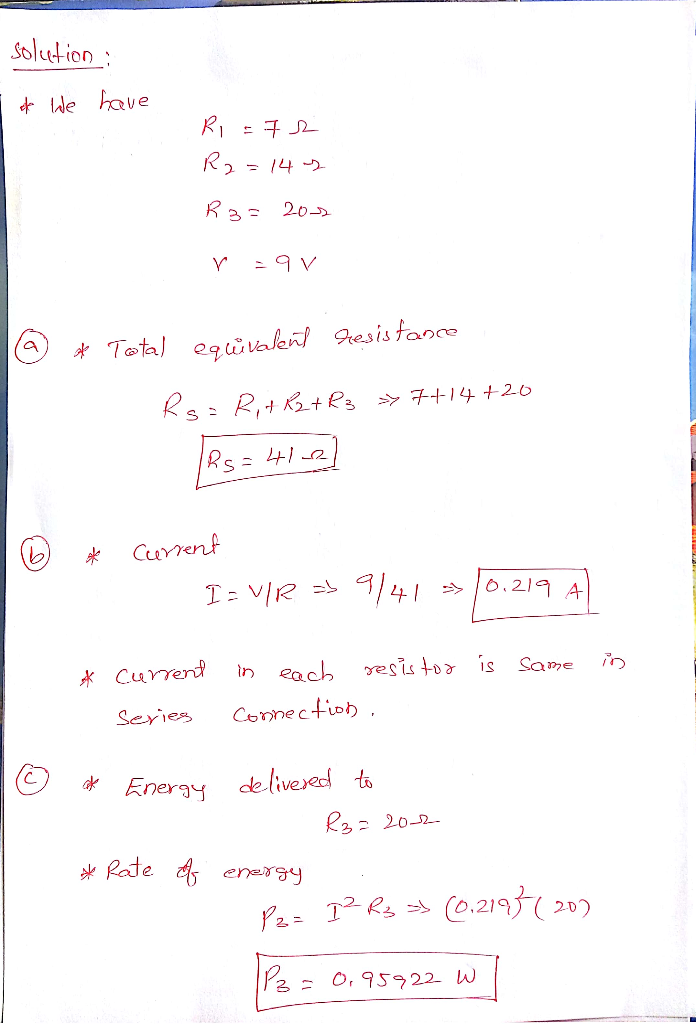#### Earn Coins

Coins can be redeemed for fabulous gifts.

Similar Homework Help Questions
• ### Three resistors with values of 4.0 Ω , 9.0 Ω , and 15 Ω are connected...

Three resistors with values of 4.0 Ω , 9.0 Ω , and 15 Ω are connected in series in a circuit with a 6.0 V battery. Part C At what rate is energy delivered to the 15 Ω resistor?

• ### Three 4.3 Ω resistors are connected in series with a 16.0 V battery. Find the following....

Three 4.3 Ω resistors are connected in series with a 16.0 V battery. Find the following. (a) the equivalent resistance of the circuit (b) the current in each resistor (c) Repeat for the case in which all three resistors are connected in parallel across the battery. equivalent resistance ______ current in each resistor _______

• ### Three 4.3 Ω resistors are connected in series with a 16.0 V battery. Find the following....

Three 4.3 Ω resistors are connected in series with a 16.0 V battery. Find the following. (a) the equivalent resistance of the circuit (b) the current in each resistor (c) Repeat for the case in which all three resistors are connected in parallel across the battery. equivalent resistance ______ current in each resistor _______

• ### Three resistors in a series connected to a battery A 2.90 Ω resistor, a 5.79 Ω...

Three resistors in a series connected to a battery A 2.90 Ω resistor, a 5.79 Ω resistor, and a 9.96 Ω resistor are connected in series with a 15.0 V battery. What is the equivalent resistance? What is the current in each resistor?

• ### Three Resistors in Parallel Three resistors are connected in parallel as shown in figure (a). A...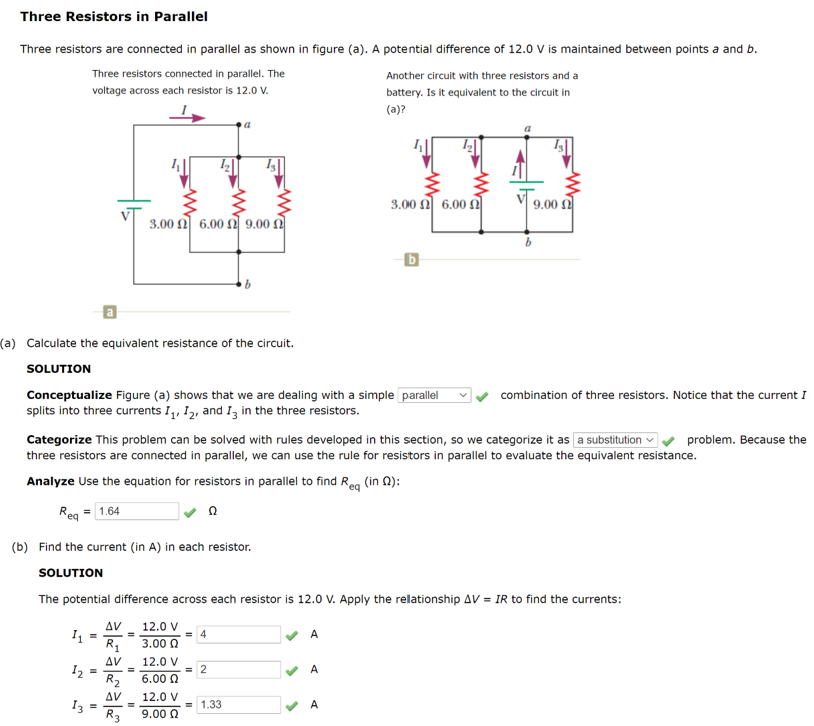Three Resistors in Parallel Three resistors are connected in parallel as shown in figure (a). A potential difference of 12.0V is maintained between points a and b. Three resistors connected in parallel. The Another circuit with three resistors and a voltage across each resistor is 12.0 V. battery. Is it equivalent to the circuit in (a)? a Ž 3.00 6.00 9.00 3.00 6.00 09.00 (a) Calculate the equivalent resistance of the circuit. SOLUTION Conceptualize Figure (a) shows that we are...

• ### 2. -/4 points (a) Three 7.80 Q resistors are connected in series to a 20.0 V...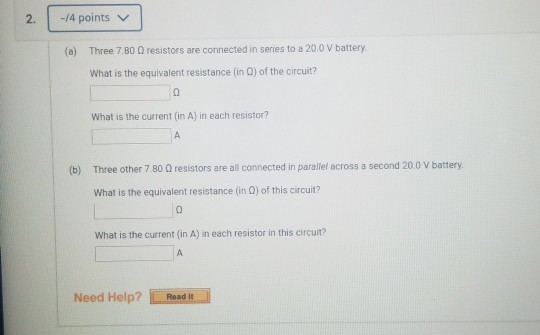2. -/4 points (a) Three 7.80 Q resistors are connected in series to a 20.0 V battery What is the equivalent resistance in o) of the circuit? What is the current in A) in each resistor? (b) Three other 7.80 Q resistors are all connected in parallel across a second 20.0 V battery What is the equivalent resistance (in) of this circuit? What is the current (in A) in each resistor in this circuit? Need Help? Read It

• ### Four resistors are connected to a battery with a terminal voltage of 12 V, as shown...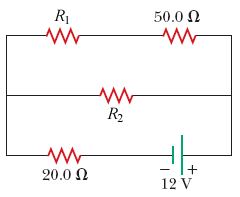Four resistors are connected to a battery with a terminal voltage of 12 V, as shown in the figure below. (Assume R1 = 31.0 Ω and R2 = 70.0 Ω.) (a) How would you reduce the circuit to an equivalent single resistor connected to the battery? Use this procedure to find the equivalent resistance of the circuit. Ω (b) Find the current delivered by the battery to this equivalent resistance. A (c) Determine the power delivered by the battery. W...

• ### Example 2: Three resistors are connected in parallel as shown. A potential difference of 18.0 V...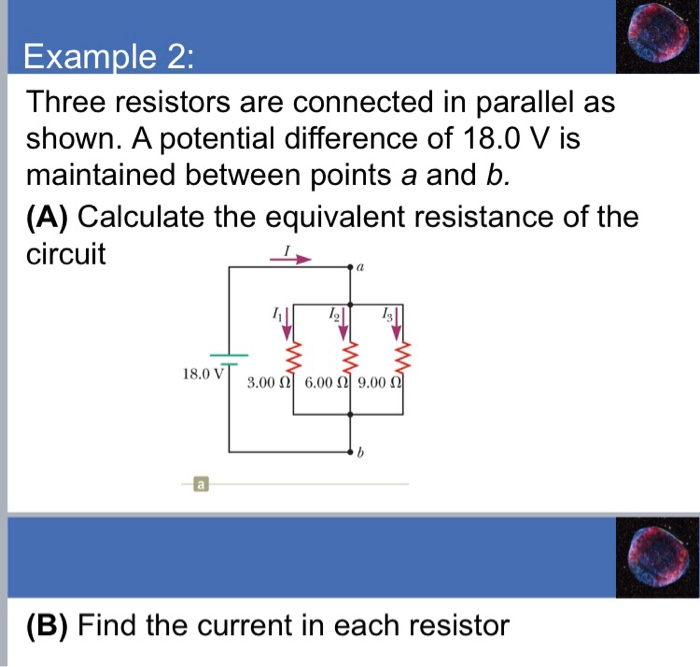Example 2: Three resistors are connected in parallel as shown. A potential difference of 18.0 V is maintained between points a and b. (A) Calculate the equivalent resistance of the circuit 18.0 V 3.00 (2] 6.00 Ω| 9.00 Ω (B) Find the current in each resistor (C) Calculate the power delivered to each resistor and the total power delivered to the combination of resistors

• ### Three resistors, 2.9 Ω, 3.2 Ω, and 5.1 Ω, are connected to a 22 V battery....

Three resistors, 2.9 Ω, 3.2 Ω, and 5.1 Ω, are connected to a 22 V battery. Find the power delivered to each resistor if they are connected (a) in series, and (b) in parallel.

• ### Three resistors (234 Ω, 159 Ω, and 301 Ω) are connected in a DC circuit. Determine...

Three resistors (234 Ω, 159 Ω, and 301 Ω) are connected in a DC circuit. Determine the equivalent resistance if: a. Each resistor is connected in series. Req = ______ Ω b. The resistors are connected in parallel to each other. Req = _______ Ω